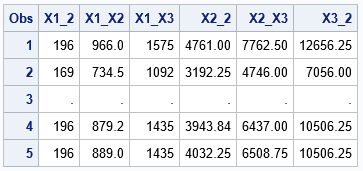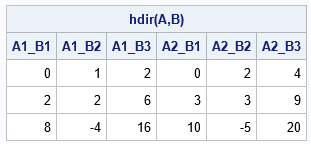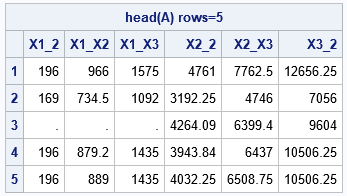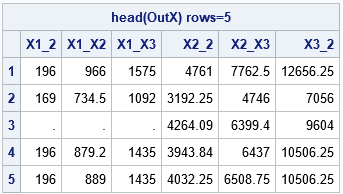I've previously written about how to generate all pairwise interactions for a regression model in SAS. For a model that contains continuous effects, the easiest way is to use the EFFECT statement in PROC GLMSELECT to generate second-degree "polynomial effects." However, a SAS programmer was running a simulation study and wanted to be able to generate all pairwise interaction effects within SAS/IML. One way to solve the programmer's problem is to use the HDIR function in SAS/IML. This article introduces the HDIR function and shows how to use it to generate pairwise (quadratic) interactions for continuous effects.

### Generate pairwise interactions by using the EFFECT statement

Before trying to solve a problem with a new tool, I like to use an existing method to solve the problem. Then I can compare the old and new answers and make sure they are the same.

For sample data, let's use the numerical variables in the Sashelp.Class data. So that the techniques are clearer, I will rename the variables to X1, X2, and X3. We want to generate all six pairwise interactions, including the "pure quadratic" terms where a variable interacts with itself. The names of the interaction effects will be X1_2, X1_X2, x1_X3, X2_2, X2_X3, and X3_2. The names with "_2" at the end are pure quadratic effects; the others are interactions.

One way to generate a design matrix that has all pairwise interactions is to use the EFFECT statement in PROC GLMSELECT. The interactions are written to the OUTDESIGN= data set. The columns of the design matrix have interpretable names, which are stored in the _GLSMOD macro variable.

```data Have; /* create the example data */ set sashelp.class(rename=(Age=X1 Height=X2 Weight=X3)); if _N_=3 then X1=.; /* add a missing value */ keep _NUMERIC_; run;   /* Use PROC GLMSELECT to generate all pairwise interactions. Step 1: Add a fake response variable */ %let DSIn = Have; /* the name of the input data set */ %let DSOut = Want; /* the name of the output data set */ data AddFakeY / view=AddFakeY; set &DSIn; _Y = 0; /* add a fake response variable */ run;   /* Step 2: Use the EFFECT statement to generate the degree-2 effects, as shown in https://blogs.sas.com/content/iml/2017/09/07/polynomial-effects-regression-sas.html */ proc glmselect data=AddFakeY NOPRINT outdesign(addinputvars)=&DSOut(drop=_Y); effect poly2 = polynomial( X1 X2 X3 / degree=2); model _Y = poly2 / noint selection=none; /* equiv to X1*X1 X1*X2 X1*X3 X2*X2 X2*X3 X3*X3 */ run; %put &=_GLSMOD; /* look at names of the interaction effects in the OUTDESIGN= data */   proc print data=&DSOut(obs=5); var X1_2 X1_X2 X1_X3 X2_2 X2_X3 X3_2; /* names are generated automatically */ run;```The output from PROC GLMSELECT contains the "pure quadratic" interactions (X1_2, X2_2, and X3_2) and the cross-variable interactions (X1_X2, X1_X3, and X2_X3). If you have k variables, there will be k pure quadratic terms and "k choose 2" cross-variable terms. Hence, the total number of quadratic interactions is "(k+1) choose 2," which is (k+1)*k/2. Here, k=3 and there are six quadratic interactions.

Notice how PROC GLMSELECT handles the missing value in the third observation: because the X1 value is missing, the procedure puts a missing value into all interaction effects.

### The horizontal direct product between matrices

The horizontal direct product between matrices A and B is formed by the elementwise multiplication of their columns. The operation is most often used to form interactions between dummy variables for two categorical variables. If A is the design matrix for the categorical variable C1 and B is the design matrix for the categorical variable C2, then HDIR(A,B) is the design matrix for the interaction effect C1*C2.

The following simple example shows how the HDIR function works. The HDIR function returns a matrix whose columns are formed by elementwise multiplication of the columns of A and the matrix B. The first set of columns is the product A[,1]#B, the second set is A[,2]#B, and so forth. If A has k1 columns and B has k2 columns, then HDIR(A,B) has k1*k2 columns.

```proc iml; /* horizontal direct product multiplies each column of A by each column of B */ A = {1 2, 2 3, 4 5}; B = {0 1 2, 1 1 3, 2 -1 4}; C = hdir(A,B); print C[c={'A1_B1' 'A1_B2' 'A1_B3' 'A2_B1' 'A2_B2' 'A2_B3'} L='hdir(A,B)'];```### Interactions of continuous variables

The previous section shows that if X is a data matrix of continuous variables, the function call HDIR(X,X) generates all pairwise combinations of columns of X. Unfortunately, the matrix HDIR(X,X) contains more columns than we need. if X contains k columns, we need the "(k+1) choose 2" =(k+1)k/2 columns of interactions, but the matrix HDIR(X,X) contains k*k columns. The problem is that HDIR(X,X) contains columns for X1*X2 and for X2*X1, even though those columns are the same. The same holds for other crossed terms such as X1*X3 and X3*X1, X2*X3 and X3*X2, and so forth.

If you want to use the HDIR function to generate all pairwise interactions, you have a choice: You can generate ALL products of pairs and delete the redundant ones, or you can compute only the unique pairs. I will show the latter because it is more efficient.

```varNames = 'X1':'X3'; use Have; read all var varNames into X; close;   /* Compute only the columns we need */ A1 = HDIR(X[,1], X[,1:3]); /* interaction of X1 with X1, X2, X3 */ A2 = HDIR(X[,2], X[,2:3]); /* interaction of X2 with X2 X3 */ A3 = HDIR(X[,3], X[,3]); /* interaction of X3 with X3 */ A = A1 || A2 || A3;   /* get the HEAD module from https://blogs.sas.com/content/iml/2021/02/10/print-top-rows-of-data-sas.html */ load module=(Head); run Head(A) colname={'X1_2' 'X1_X2' 'X1_X3' 'X2_2' 'X2_X3' 'X3_2'};```The HEAD module displays only the top five rows of the matrix of interactions. This output is almost the same as the output from PROC GLMSELECT. A difference is how missing values propagate. For the third row, X1 is missing. PROC GLMSELECT puts a missing value in all columns of the pairwise interactions. In contrast, the SAS/IML output only has missing values in the first three columns because those are the only columns that involve the X1 variable. If your data contain missing values, you might want to use the CompleteCases function to delete the rows that have missing values before performing additional analyses.

### A function that computes interactions of continuous variables

The previous section computes all quadratic interactions for a matrix that has three variables. It is straightforward to generalize the idea to k variables. You simply compute the interactions HDIR(X[,i],X[,i:k]) for i=1,2,...,k. This is done in the following program. During the computation, it is convenient to also generate names for the interactions. The following program generates the same names as PROC GLMSELECT. To make the code easier to understand, I encapsulate the logic for names into a helper function called GetNamePairs. The helper function is called as part of the InteractPairs module, which returns both the matrix of interactions and the character vector that names the columns:

```/* Helper function: Form names of interaction effects. Input: s is a scalar name. t is a character vector of names. Return row vector of pairs of names "s_2" or "s_t[i]" Example: GetNamePairs('X1', 'X1':'X3') returns {'X1_2' 'X1_X2' 'X1_X3'} */ start GetNamePairs(s, t); k = nrow(t)*ncol(t); b = blankstr(nleng(s)+nleng(t)+1); /* max length of interaction name */ pairs = j(1, k, b); do i = 1 to k; if s=t[i] then pairs[i] = strip(s) + "_2"; else pairs[i] = catx("_", strip(s), strip(t[i])); end; return pairs; finish;   /* Generate all quadratic interactions for continuous variables. Input: design matrix X and a vector of column names. Output: OutX: a matrix whose columns are the pairwise interactions OutNames: the names of interactions, such as Age_2 and Height_Age */ start InteractPairs(OutX, OutNames, X, Names); k = ncol(X); numInteract = comb(k+1,2); /* = k + comb(k,2) */ OutX = j(nrow(X), numInteract, .); OutNames = j(1, numInteract, BlankStr(2*nleng(Names)+1)); col = 1; /* initial column to fill */ do i = 1 to k; m = k-i+1; /* number of interaction for this loop */ OutX[,col:col+m-1] = HDIR(X[,i], X[,i:k]); OutNames[,col:col+m-1] = GetNamePairs(Names[i], Names[i:k]); col = col + m; end; finish;   run InteractPairs(OutX, OutNames, X, varNames); run Head(OutX) colname=OutNames;```The output is identical to the earlier output. The input arguments to this module can have an arbitrary number of columns. In this example, the design matrix does not include an intercept column (a column that is all ones). Consequently, the output is the set of all quadratic interactions. If your design matrix includes an intercept column, the output will contain an intercept column and all main effects in addition to the quadratic effects.

Technically, you don't need to use the HDIR function in the InteractPairs module. Instead, you can use the familiar '#' operator to perform the elementwise multiplication. However, if you try to generalize the module to handle the interaction between categorical variables and continuous variables, the HDIR function will be useful.

### Summary

This article introduces the HDIR function in SAS/IML, which computes the horizontal direct product of matrices. You can use the HDIR function to generate interaction effects in regression models. This article shows how to generate all quadratic interactions among a set of continuous variables.

The post Generate all quadratic interactions in a regression model appeared first on The DO Loop.